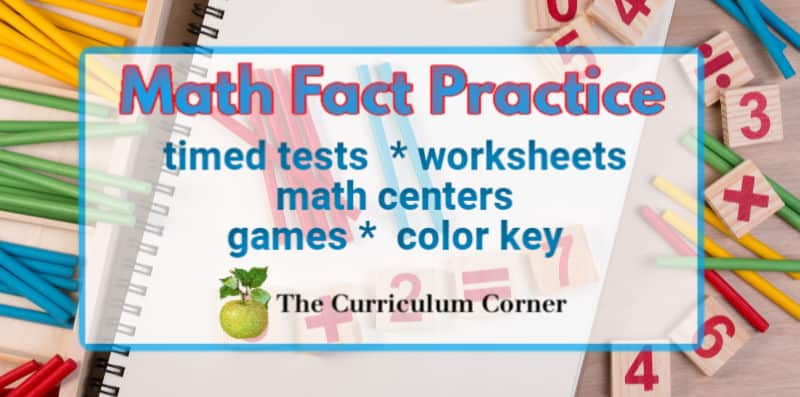# Math Facts

### The Curriculum Corner is your first stop for math fact practice in the classroom and at home!Our free math fact practice resources include timed tests, worksheets, math centers, computation games and color key pages.

This is a great place for teachers and parents to begin gathering classroom and home resources.

With the help of the provided materials, you will be able to help your students work on mastering addition, subtraction, multiplication and division facts.

### Why is math fact mastery important?

There are often debates about the need for students to master basic facts. With calculators, phones and tablets readily available to students, many question the need for students to spend time memorizing addition, subtraction, multiplication and division facts.

We believe that math fact mastery can benefit students and is worth the time required.

### Benefits of math fact mastery:

Mastery of basic skills make higher level mathematics easier in the future. Students can spend time grasping new concepts instead of using the time to solve basic facts.

Math fact mastery can also benefit students in subjects other than math. For example, when a formula is being solved in science, not knowing facts could lead to an incorrect solution.

Confidence! Students know when others can quickly give answers and they have to struggle. Helping children develop mastery of math facts can help them build confidence in their math skills.

### How long should students be given to solve each problem?

So often we are asked how many math facts students should be able to solve in a minute.

We have looked into this and the most common answer we find is that students should be able to answer 20 problems in 1 minute.

Or, this means that students should be allowed 3 seconds per fact.

As always, we encourage you to look at the individual students and their needs. While 3 seconds per fact is the standard, it’s always important to make sure this is an appropriate standard for each child.

## True/False Equation Sorts

Download this true / false equation sort for addition and subtraction fact practice in your first or second grade classroom. This is another free resource for teachers and homeschool families from The Curriculum Corner.